# Test: Control System- 4

## 25 Questions MCQ Test Basic Electronics Engineering for SSC JE (Technical) | Test: Control System- 4

Description
Attempt Test: Control System- 4 | 25 questions in 100 minutes | Mock test for Electrical Engineering (EE) preparation | Free important questions MCQ to study Basic Electronics Engineering for SSC JE (Technical) for Electrical Engineering (EE) Exam | Download free PDF with solutions
QUESTION: 1

Solution:
QUESTION: 2

Solution:
QUESTION: 3

### How many number of branches the roots loci of the equation (s+20 (s+3)+ K (s+1) =0 Have ?

Solution:
QUESTION: 4

The characteristic equation of a control system is given as
s4+ 4s3 + 4s2 + 3s + K = 0
What is the value of K for which this system is marginally stable ?

Solution:
QUESTION: 5

The transfer function of a controller is given as

kp + kd.s where Kp and Kd are constant.

What type of controller is this ?

Solution:
QUESTION: 6

The transfer function of a controller is given as

kp + Kd.s + Ki/s where Kp, Kd and Ki are constant.

What types of controller is this ?

Solution:
QUESTION: 7

A linear time-invariant system initially at rest, when subjected to a unit -step input, gives a response y(t) = te- t > 0 . The transfer function of the system is :

Solution:
QUESTION: 8

If the gain of the system is reduced to zero value, the roots of the system in the s-plane

Solution:
QUESTION: 9

The addition of open loop zero pulls the root-loci towards

Solution:
QUESTION: 10

In the Bode plot of a unity feedback control system, the value of phase angle of G(jw) is -90º at the gain cross over frequency of the bode plot, the phase margin of the system is

Solution:
QUESTION: 11

The Nyquist plot of loop transfer function G(s) H(s) of a closed loop control system passes through the point (–1, j0) is the G(s) H(s) plane. The phase margin of the system is

Solution:
QUESTION: 12

The transfer function G(s) =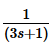has a corner frequency is

Solution:
QUESTION: 13

The transfer function for the state representation of continuous time LTI system :

q(t) = Aq(t) + b×(t)

y(t) = cq(t) + d×(t) is given by

Solution:
QUESTION: 14

Where are the K=0 point on the root loci of the characteristic equation of the closed loop control system located at ?

Solution:
QUESTION: 15

If the gain margin of a system in decibedls is negative, the system is

Solution:
QUESTION: 16

An electrical network shown in figure. What type of compensator in this ?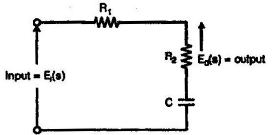Solution:
QUESTION: 17

The circuit diagram of an electrical network is given in figure. What type of compensator is this?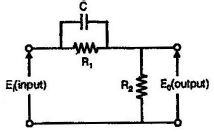Solution:
QUESTION: 18

Given a unity feedback system with G(s) =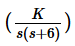,the value of K for damping ratio of 0.75 is

Solution:
QUESTION: 19

System is said to be marginally stable, if

Solution:
QUESTION: 20

The type of system which is used for determination of static error constants is determination from the number of

Solution:
QUESTION: 21

The time taken for the output to settle within + 2% of step input for the control system represented by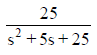is given by

Solution:
QUESTION: 22

The effect of integral controller on the steady state error (ess) and the relative stability (Rs) of the system are

Solution:
QUESTION: 23

A proportional integral (P|) controller results in which of the following ?

Solution:
QUESTION: 24

A Phase lead compensating network has its transfer function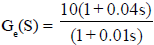The maximum phase lead occurs at a frequency of

Solution:
QUESTION: 25

A system is described by the transfer function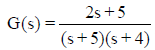The dc gain of the system is

Solution:Use Code STAYHOME200 and get INR 200 additional OFF Use Coupon Code